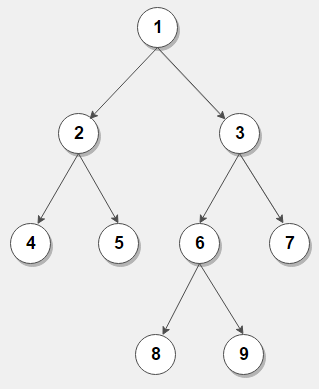# Print all paths from leaf to root node in a binary tree

Given a binary tree, write an recursive algorithm to print all paths from leaf to root node in a binary tree.

For example, consider below binary treeThere are five leaf to root path in above binary tree –

4 -> 2 -> 1
5 -> 2 -> 1
8 -> 6 -> 3 -> 1
9 -> 6 -> 3 -> 1
7 -> 3 -> 1

The idea is to traverse the tree in preorder fashion and store every encountered node in current path from root to leaf in a vector. If we encounter a leaf node, we print all nodes present in the vector in reverse order.

Here’s a C++ program that demonstrates it:

Output:

4 -> 2 -> 1
5 -> 2 -> 1
8 -> 6 -> 3 -> 1
9 -> 6 -> 3 -> 1
7 -> 3 -> 1

The time complexity of above solution is O(n) and need O(h) extra space for the call stack where h is the height of the tree.

Exercise:

1. Write iterative implementation of above problem.

2. Modify the solution to print leaf to root path having sum of nodes equal to a given number.(1 votes, average: 5.00 out of 5)Loading...

Please use our online compiler to post code in comments. To contribute, get in touch with us.
Like us? Please spread the word and help us grow. Happy coding 🙂Subscribe
Notify ofGuest
TheDarkKnight

Please share the iterative approach.Guest

Instead of copy vector evetytime, its better we send a reference and pop the element after both left and right are done
void inorderRecursive(Node *root, vector &path) {
if (root == NULL) return;
if (root->left == NULL && root->right == NULL) {

for(auto i : path) {
cout << i << " ";
}
cout <data <data);
inorderRecursive(root->left, path);
inorderRecursive(root->right, path);
path.pop_back();
}Guest

The linked question was Root to Leaf, but this page discuss about Leaf to Root
https://www.techiedelight.com/list-of-problems/
Question #27